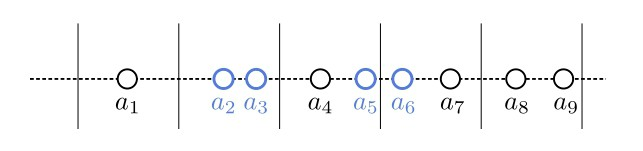## 1. Introduction

In this tutorial, we’ll show how to find the two numbers with the minimum absolute difference in an array.

## 2. The Minimal Difference

We have a numerical arrayand want to find the two closest numbers.

For example, if, the minimal absolute difference is betweenand, so our algorithm should output the pair.

## 3. Solution

Let’s suppose that we’ve sortedin the non-decreasing order:. Then, the closest number to eachis immediately before or right after it. In other words, we need to check only the differences between the consecutive elements if the array is sorted (the order doesn’t matter).

So, we can find the minimal difference by iterating over the sortedand computingfor each. For instance, sorting, we get. The absolute differences between consecutive elements are, respectively. Therefore, the answer is the second pair:.

### 3.1. Pseudocode

Here’s the pseudocode:First, we sort the array. Then, we declare the first pairas the current closest pair and loop over the rest. We update the current closest pair if we find two consecutive numbers with a smaller absolute difference.

### 3.2. Complexity

What’s the time complexity of this approach? It depends on the sorting algorithm because the search for the minimal difference in the sorted array isin the worst and average cases. With Merge Sort, the sorting part takestime in the worst case, so the entire algorithm’s complexity is.

If we used Quicksort, whose worst-case time complexity is, our approach would also be quadratic in the worst case. However, Quicksort is log-linear in the average case and has a smaller multiplicative coefficient than the Merge Sort. For that reason, pairing the linear search with Quicksort instead of Merge Sort may work faster in practice, although the latter is asymptotically more favorable.

Now, the question is: can we do better?

## 4. Rabin’s Algorithm in One Dimension

And the answer is that we can, at least when it comes to probabilistic time complexity. In 1976, Michael O. Rabin formulated a randomized algorithm for finding the closest pair inand proved it achieved a linear time complexity with high probability. For, the closest-pair problem reduces to ours.

### 4.1. The Idea

The main idea is as follows. First, we samplepairsof numbers from() and compute the minimal difference between the pairs. Let’s denote it as. Afterward, we sort all the numbers into consecutive segments of length.

Then, the closest two numbers are either in the same segment or in two neighboring ones:So, we iterate over the segments and find the closest pairs in each segment and every two consecutive ones, keeping track of the minimal difference calculated. In the end, it corresponds to the actual minimum difference in.

### 4.2. Pseudocode

Here’s the pseudocode:First, we samplepairs and determine the closest one. Letbe the absolute difference between those two numbers. Then, we divide the array into-wide segments (let’s suppose there areof those). We can determine the segment into whichfalls by rounding down the quotient. So, we keep the numbers that belong to the same segment in the same entry of, a dictionary whose integer keys identify the-wide segments, and the values are arrays (lists or trees) of the corresponding elements.

Afterward, we loop over the keys inin the sorted order. The closest two numbers in the entire array are either in the same segment or two consecutive ones. So, looping over, we compare the current closest pairto the closest one in each. Ifexists, we compareto the two numbers such that one belongs toand the other to.

### 4.3. Time Complexity

This algorithm has a linear expected time complexity with high probability, even if we use the quadratic brute-force approach to find the closest pairs in individual segments.

## 5. Conclusion

In this article, we showed how to find the closest two numbers in an array. We presented two algorithms: one based on sorting and the other randomized. The former is log-linear in the worst case if we use Merge Sort, but the latter achieves a linear expected time with high probability.